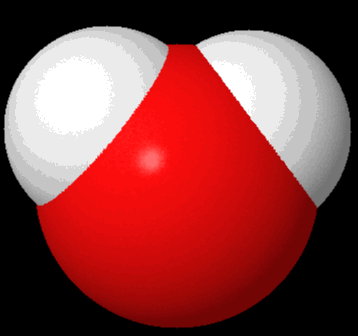# Surface Area of a Molecule

Geometry Level 5A new molecule $\ce{A2Z}$ has been discovered. It is made of two atoms of $\ce{A}$ and one atom of $\ce{Z}$. The surface area of a molecule can tell us loads about the chemical and physical properties of the molecule, hence it is imperative that we calculate it.

If the surface area of an $\ce{A2Z}$ molecule is $S \text{ pm}^2$, then what is $\dfrac{S}{\pi}$?

Details and Assumptions

• We can model the molecule as three overlapping spheres as shown in the animation. The white spheres are atoms of $\ce{A}$ and the red sphere is an atom of $\ce{Z}$

• The radius of Sphere $\ce{A}$ is 40 pm

• The radius of Sphere $\ce{Z}$ is 60 pm

• The distance between the centers of Sphere $\ce{A}$ and Sphere $\ce{Z}$ is 80 pm

×